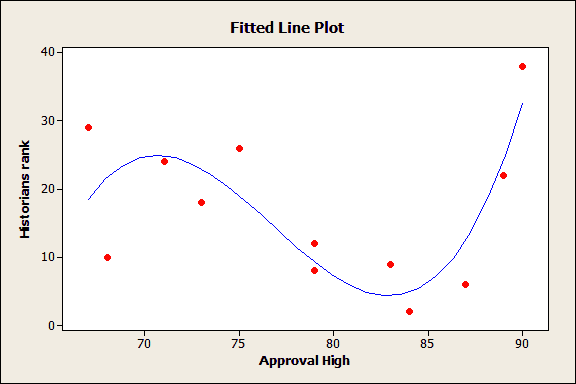Topics: Regression Analysis, StatisticsIn regression analysis, overfitting a model is a real problem. An overfit model can cause the regression coefficients, p-values, and R-squared to be misleading. In this post, I explain what an overfit model is and how to detect and avoid this problem.

An overfit model is one that is too complicated for your data set. When this happens, the regression model becomes tailored to fit the quirks and random noise in your specific sample rather than reflecting the overall population. If you drew another sample, it would have its own quirks, and your original overfit model would not likely fit the new data.

Instead, we want our model to approximate the true model for the entire population. Our model should not only fit the current sample, but new samples too.

The fitted line plot illustrates the dangers of overfitting regression models. This model appears to explain a lot of variation in the response variable. However, the model is too complex for the sample data. In the overall population, there is no real relationship between the predictor and the response. You can read about the model here.

## Fundamentals of Inferential Statistics

To understand how overfitting causes these problems, we need to go back to the basics for inferential statistics.

The overall goal of inferential statistics is to draw conclusions about a larger population from a random sample. Inferential statistics uses the sample data to provide the following:

• Unbiased estimates of properties and relationships within the population.
• Hypothesis tests that assess statements about the entire population.

An important concept in inferential statistics is that the amount of information you can learn about a population is limited by the sample size. The more you want to learn, the larger your sample size must be.

You probably understand this concept intuitively, but here’s an example. If you have a sample size of 20 and want to estimate a single population mean, you’re probably in good shape. However, if you want to estimate two population means using the same total sample size, it suddenly looks iffier. If you increase it to three population means and more, it starts to look pretty bad.

The quality of the results worsens when you try to learn too much from a sample. As the number of observations per parameter decreases in the example above (20, 10, 6.7, etc), the estimates become more erratic and a new sample is less likely to reproduce them.

## Applying These Concepts to Overfitting Regression Models

In a similar fashion, overfitting a regression model occurs when you attempt to estimate too many parameters from a sample that is too small. Regression analysis uses one sample to estimate the values of the coefficients for all of the terms in the equation. The sample size limits the number of terms that you can safely include before you begin to overfit the model. The number of terms in the model includes all of the predictors, interaction effects, and polynomials terms (to model curvature).

Larger sample sizes allow you to specify more complex models. For trustworthy results, your sample size must be large enough to support the level of complexity that is required by your research question. If your sample size isn’t large enough, you won’t be able to fit a model that adequately approximates the true model for your response variable. You won’t be able to trust the results.

Just like the example with multiple means, you must have a sufficient number of observations for each term in a regression model. Simulation studies show that a good rule of thumb is to have 10-15 observations per term in multiple linear regression.

For example, if your model contains two predictors and the interaction term, you’ll need 30-45 observations. However, if the effect size is small or there is high multicollinearity, you may need more observations per term.

## How to Detect and Avoid Overfit Models

Cross-validation can detect overfit models by determining how well your model generalizes to other data sets by partitioning your data. This process helps you assess how well the model fits new observations that weren't used in the model estimation process.

Minitab statistical software provides a great cross-validation solution for linear models by calculating predicted R-squared. This statistic is a form of cross-validation that doesn't require you to collect a separate sample. Instead, Minitab calculates predicted R-squared by systematically removing each observation from the data set, estimating the regression equation, and determining how well the model predicts the removed observation.

If the model does a poor job at predicting the removed observations, this indicates that the model is probably tailored to the specific data points that are included in the sample and not generalizable outside the sample.

To avoid overfitting your model in the first place, collect a sample that is large enough so you can safely include all of the predictors, interaction effects, and polynomial terms that your response variable requires. The scientific process involves plenty of research before you even begin to collect data. You should identify the important variables, the model that you are likely to specify, and use that information to estimate a good sample size.

For more about the model selection process, read my blog post, How to Choose the Best Regression Model. Also, check out my post about overfitting regression models by using too many phantom degrees of freedom. The methods described above won't necessarily detect this problem.